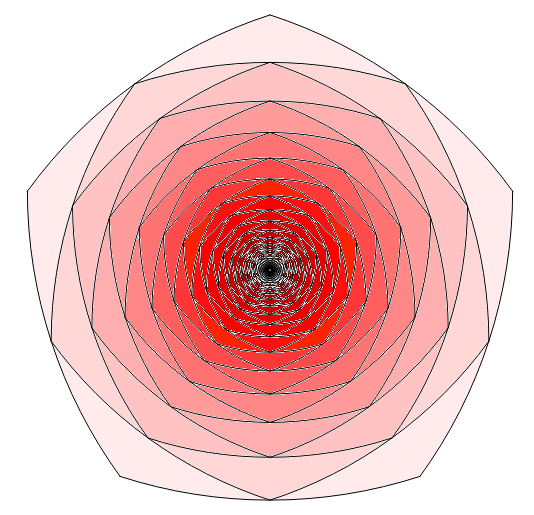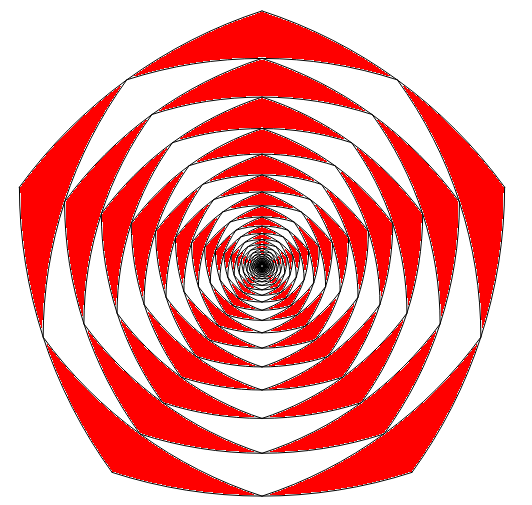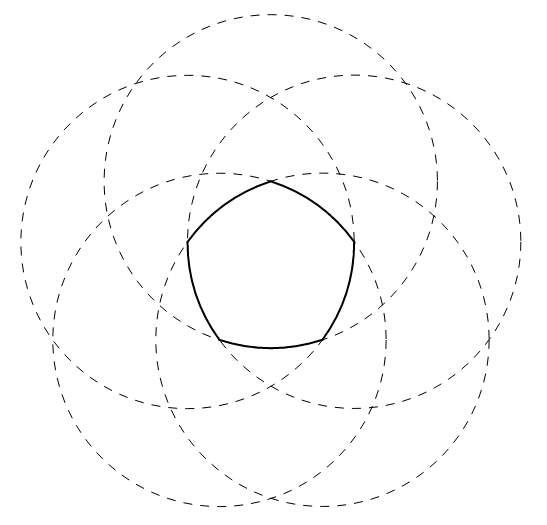# Reuleaux Rosie

Geometry Level 5Reuleaux polygon done by Geogebra

While Mai and Joey were preparing for the party, Tea Gardner and Yugi Muto, both lovers, hung out at the church, where they stared at a stain-glassed Reuleaux pentagon window, filled with pink and red colors. The conversation started:

Tea: Wow! What a beautiful rose! Isn't it beautiful, Yugi?

Yugi: Yes, it is!

Tea: I have a math problem for you to try out. Suppose we start with five unit circles, where each center is intersected by two circular arcs. Then, this makes a Reuleaux pentagon.

Yugi: That is easy to remember...

Tea: But there's more! Draw another Reuleaux pentagon inside, where each vertex intersects the midpoint of the circular arcs. Repeat this infinitely, so we have much like the stain-glassed one. Here is the following:

[After minutes of perfect-sketching]This is the main diagram to focus for the problem.

Here is the rose with alternating red and white petals. Your goal is to compute the area of all red petals.

Yugi: Touche! What a long geometry problem you asked here! Is there a specific formula to generalize the area of the red petals after finite number of iterations?

Tea: If I give that away, then you will know the answer to this fun problem! Solve this correctly, and we will enjoy a nice party! :)

What is the area of all red petals in the second diagram, where the diagonal of the largest Reuleaux pentagon is 1? If your area is $A$, input $\left\lfloor A \cdot 10^3 \right\rfloor$ as your answer.

PreliminariesA Reuleaux polygon is a curvilinear polygon formed by an odd number of circular arcs. Like a Reuleaux triangle, the apex points of a Reuleaux polygon are centers of identical circles.

For the main problem, determine the area of a Reuleaux pentagon.

Bonus: Generalize this for any odd number of vertices and circular arcs. You should notice that as the number of edges approaches $\infty$, a Reuleaux polygon becomes close to a circle of area $\frac{\pi}{4}r^2$, where $r$ is the radius of the large circle.

This is the end of the fourth chapter of the story. Check chapter directory if you are interested:

First - Second - Third - Fourth - Fifth

×